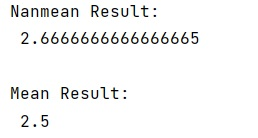# NumPy's mean() and nanmean() Methods

Learn, how to find the IQR in NumPy in Python?
Submitted by Pranit Sharma, on January 12, 2023

NumPy is an abbreviated form of Numerical Python. It is used for different types of scientific operations in python. Numpy is a vast library in python which is used for almost every kind of scientific or mathematical operation. It is itself an array which is a collection of various methods and functions for processing the arrays.

## numpy.mean() and numpy.nanmean() methods

Both methods are used to calculate the mean of the values of the numpy array. The numpy.mean() computes the arithmetic mean along the specified axis. It returns the average of the array elements. The average is taken over the flattened array by default, otherwise over the specified axis. float64 intermediate and return values are used for integer inputs.

While on the other hand, the numpy.nanmean() computes the arithmetic mean along the specified axis, ignoring NaNs.

Let us understand with the help of an example,

## Python code to demonstrate the example of NumPy's mean() and nanmean() Methods

```# Import numpy
import numpy as np

# Creating numpy array
arr = np.array([[1, np.nan], [3, 4]])

# Using nanmean method
res = np.nanmean(arr)

# Display nan mean result
print("Nanmean Result:\n",res,"\n")

# Creating array for mean
arr = np.array([[1, 2], [3, 4]])

# Using mean
res1 = np.mean(arr)

# Display mean result
print("Mean Result:\n",res1,"\n")
```

Output:What's New (MCQs)

Top Interview Coding Problems/Challenges!

IncludeHelp's Blogs

Languages: » C » C++ » C++ STL » Java » Data Structure » C#.Net » Android » Kotlin » SQL
Web Technologies: » PHP » Python » JavaScript » CSS » Ajax » Node.js » Web programming/HTML
Solved programs: » C » C++ » DS » Java » C#
Aptitude que. & ans.: » C » C++ » Java » DBMS
Interview que. & ans.: » C » Embedded C » Java » SEO » HR
CS Subjects: » CS Basics » O.S. » Networks » DBMS » Embedded Systems » Cloud Computing
» Machine learning » CS Organizations » Linux » DOS
More: » Articles » Puzzles » News/Updates